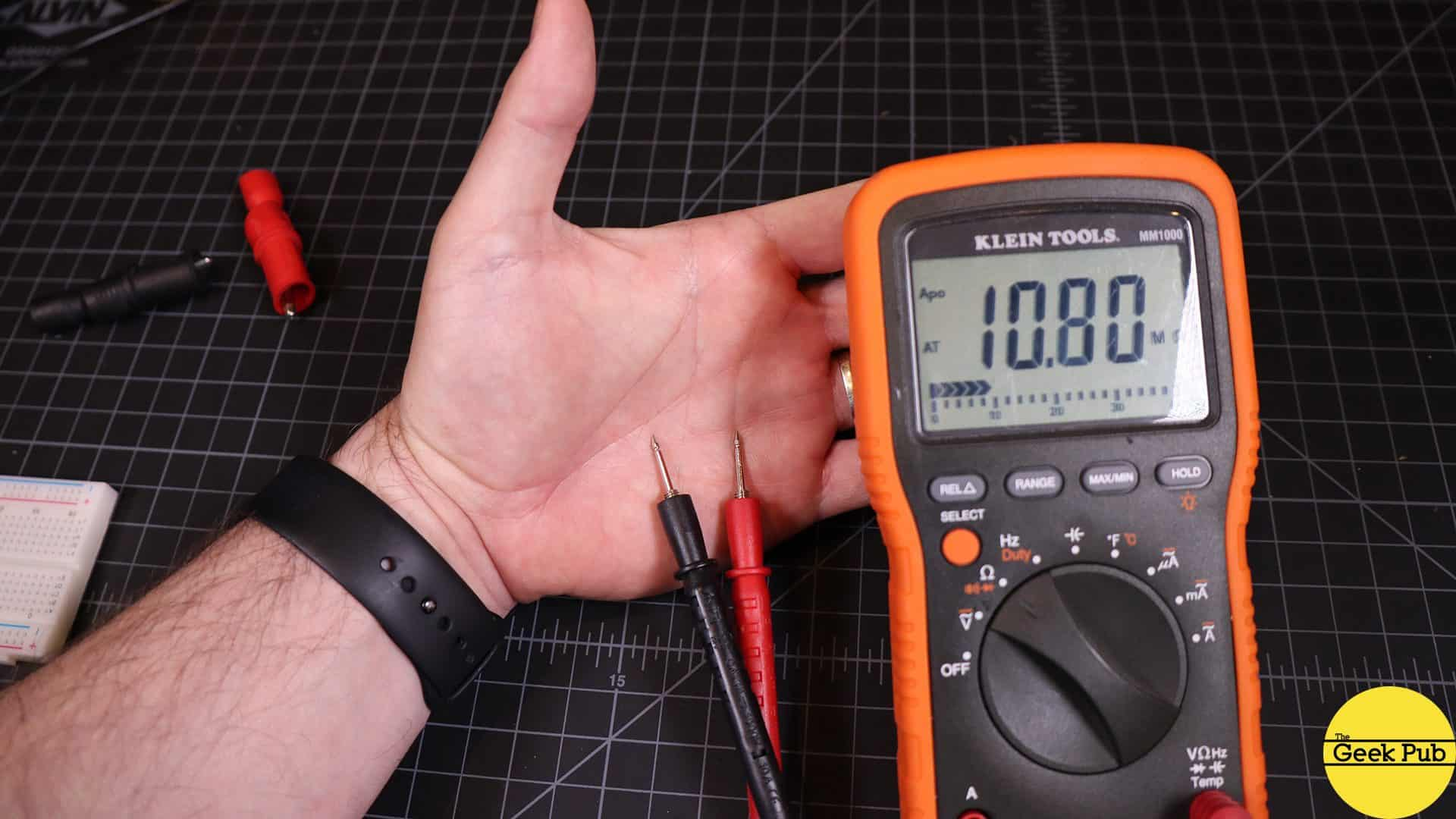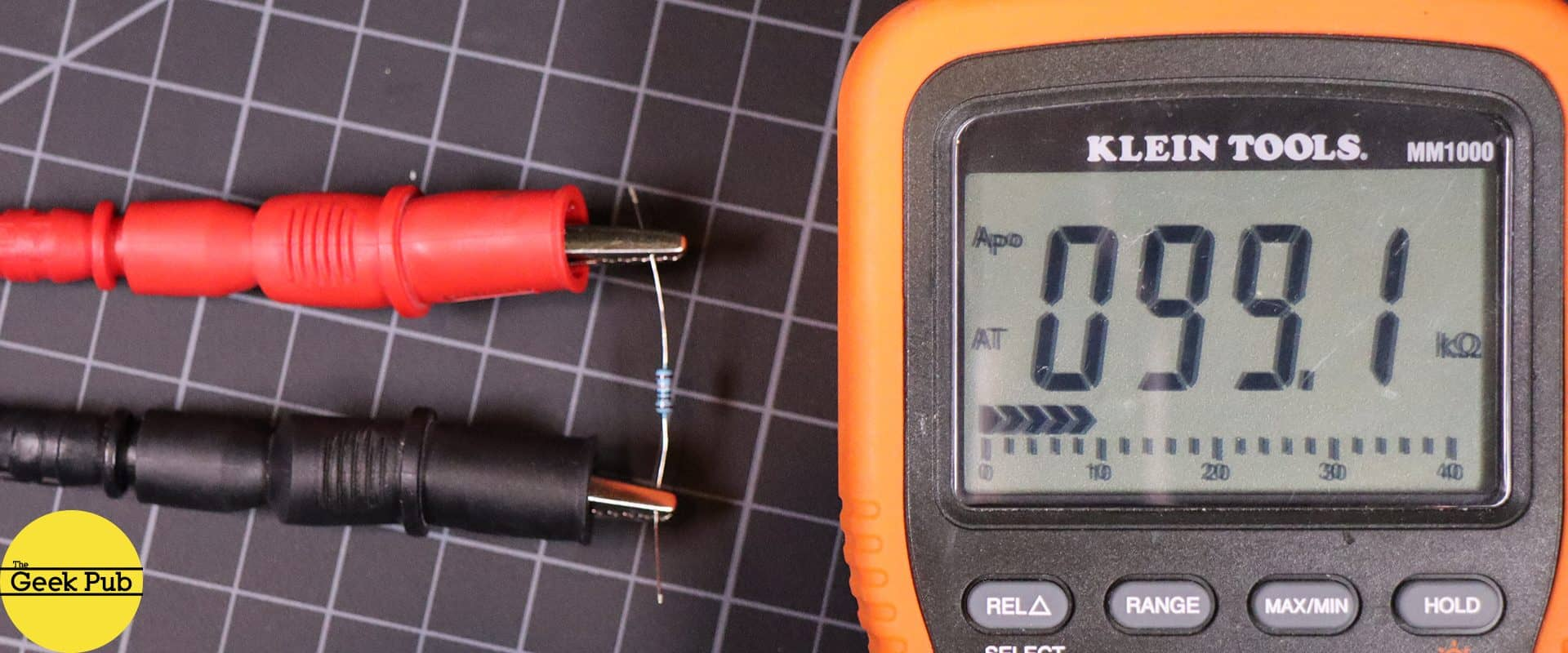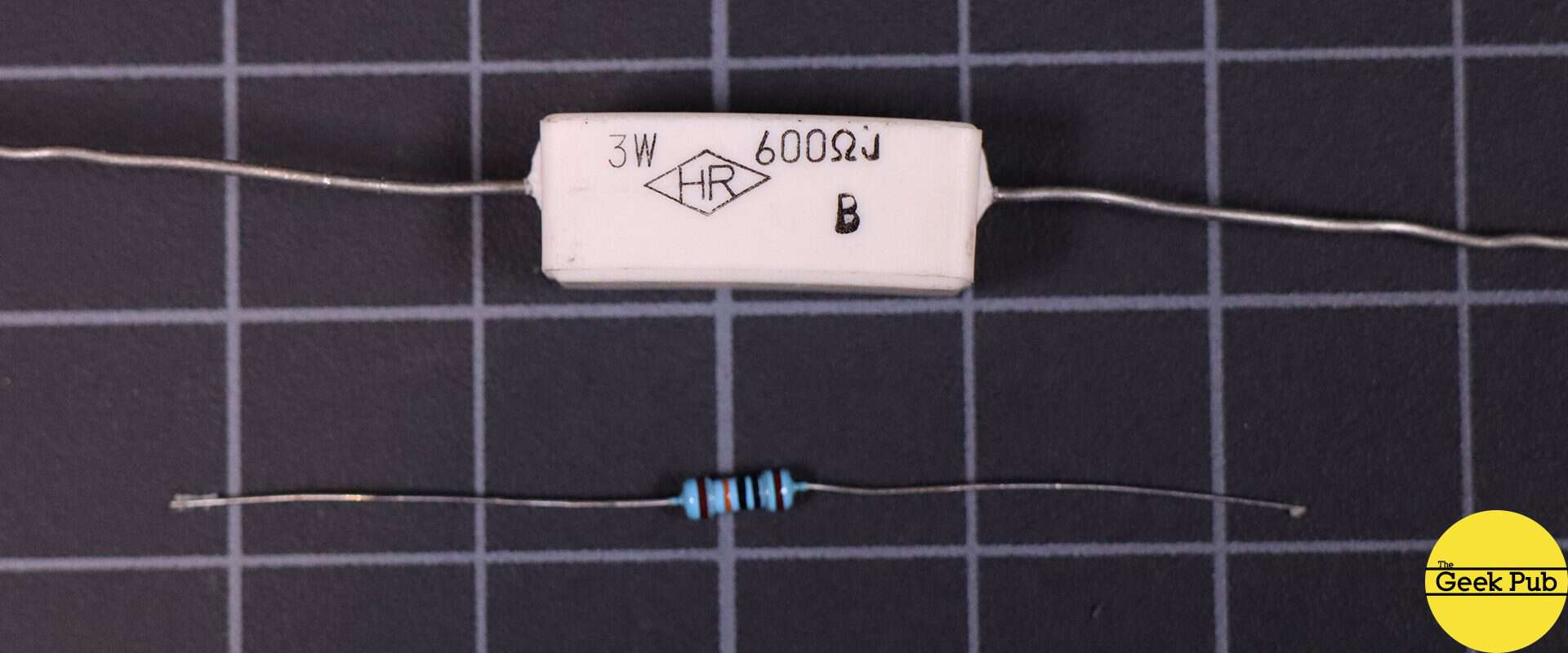You’ve probably seen resistors in most of the projects we do here at The Geek Pub, and wondered to yourself “What is resistance?”.  Well today we’re going to do a tutorial on resistors and learn exactly what resistance is and how it works!

## How Resistance Works

In our voltage tutorial we discussed how voltage seems to behave like a pushing force, that pushes electrons from atom to atom through a wire creating an electric circuit. It turns out that this process is not 100% efficient!  The atoms in a copper wire are always vibrating around just a small amount due to the heat energy in them and when electrons try to move through the wire bouncing from atom to atom, sometimes they’ll bump into an atom that’s in the way. The movement of that electron gets “resisted”.

RELATED: How Current Works

That resistance is turned into heat, and in fact is the basic principle behind the electric heaters you find at most big box stores, as well as the filaments in tried and true incandescent light bulbs that hopefully you’ve swapped out for LEDs by now!  Instead of copper wire they use a filament wire that has a higher resistance to the flow of electrons!

Metals are generally considered to be very low resistance materials and due to this they make great conductors of electricity. There are many materials however that do not.  Most resistors are made out of a metal-oxide material that is designed to resist the flow of current at a specific value or “Ohm”.If you’ve not read our tutorials on voltage and current, the basic principles for this tutorial can be explained as follows:

• Voltage is the difference in charge (or potential energy) between two points
• Current is the rate at which that charge flows
• Resistance is a material’s tendency to resist the flow of that charge (or current).

## What is Ohm’s Law?

Resistance is measured in a unit called Ohms, which is represented by the Greek letter Omega (Ω).

Ohm’s law states that “the current through a conductor between two points is directly proportional to the voltage across the two points.”

We talk about resistors in Ohms. A resistor might be a 220 Ohm resistor, or it might be 6.8K Ohm resistor.  To give you a frame of reference, something with an Ohm value of 1 or less is considered to be a very low resistance.  Most wire you use in your projects will fit in this category. A 1 megaohm resistor (or 1 million Ohms) is a very high level of resistance and almost no current will pass through.

You can see here that the resistance of my human skin is about 10 megaohms!Sometimes you need to find the correct resistance value to limit current. The formula for calculating resistance is: R = E ÷ A

In this algebraic expression, Resistance (R) is equal to current (A) divided by voltage (I). As with any algebraic expression, you can find the answer to any one of these variables as long as you have the other two.

## How to Measure Resistance and Read the Color Codes

Next up as we continue to answer the question “What is resistance?”, we;re going to learn how to measure resistance and read the color codes on resistors.

The best way to measure resistance is to use a multimeter. If you don’t have a multimeter we have a multimeter tutorial and buying guide you can reference.  Mutimeters offer a function for measuring Ohms by touching their probes to each end of a resistor.Most resistors have colored bands on them that identify their value and some other important information about their specifications.

This code can be read if you have a decoder, which we’ve created a hand one for you below. The colors translate into resistance values, and accuracy tolerances. Some people choose to memorize these color codes, but my brain just can’t seem to do it. So I always go back to this handy resistor code chart.  We also have a very handy online resistor calculator you can use.These types of standard resistors are perfect for most projects you’ll do at home and are usually rated for 1/4 or 1/2 watt.  Sometimes you need bigger capacity resistors for projects like amplifiers and power supplies. Resistors come in all kinds of shapes, sizes and specifications.  Check out this 3 watt resistor compared to a 1/4 watt resistor!Resistors kits are available on Amazon for just a few bucks!